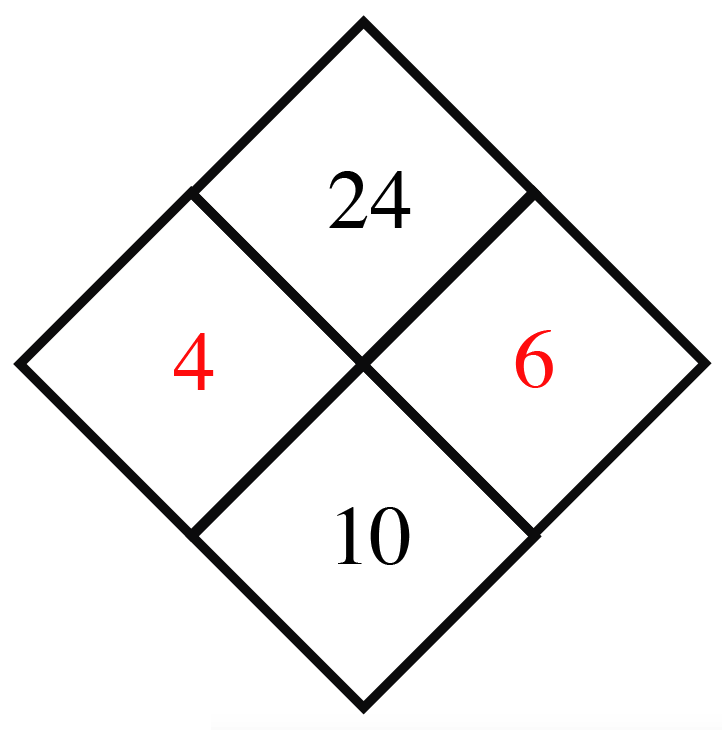### Home > CC2MN > Chapter 4 > Lesson 4.2.1 > Problem4-32

4-32.

Copy and complete each of the Diamond Problems below. The pattern used in the Diamond Problems is shown at right.1.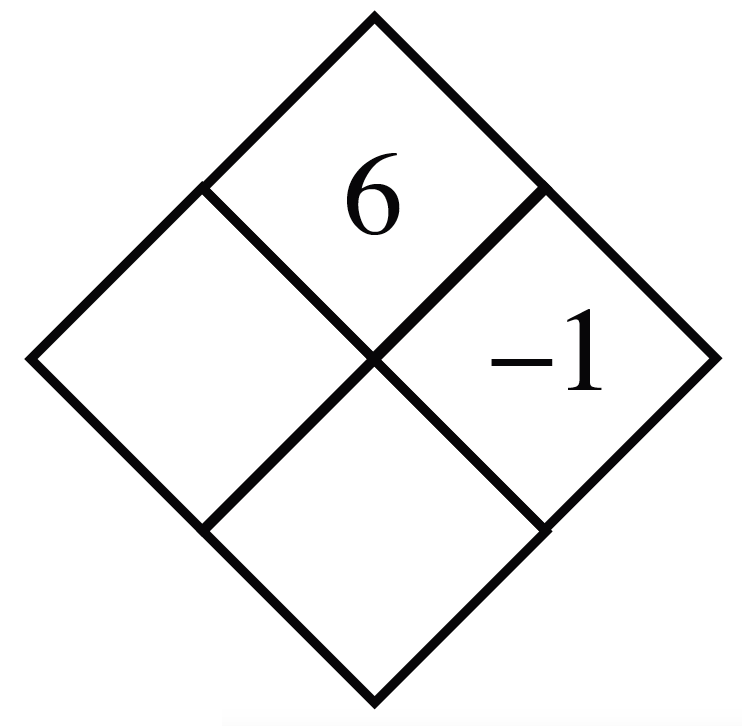Divide $6$ by $-1$.
$6\div\left(-1\right)=-6$

Add $-6$ to $-1$.
$(-6)+(-1)=-7$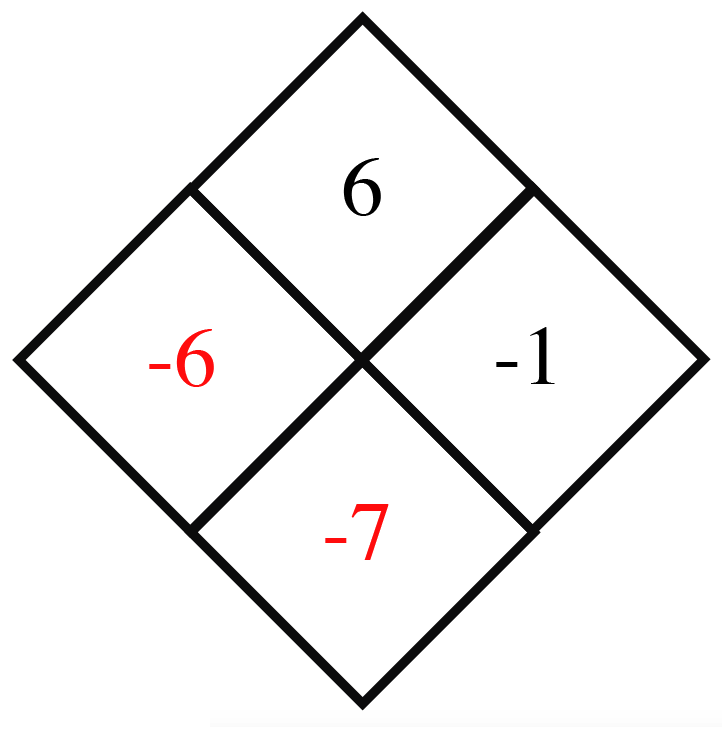1.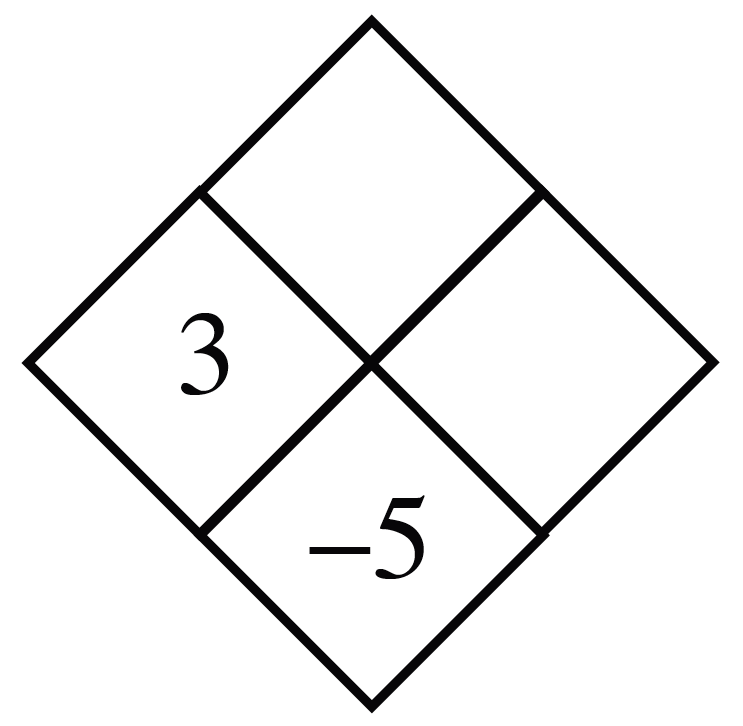$3+?=-5$

1.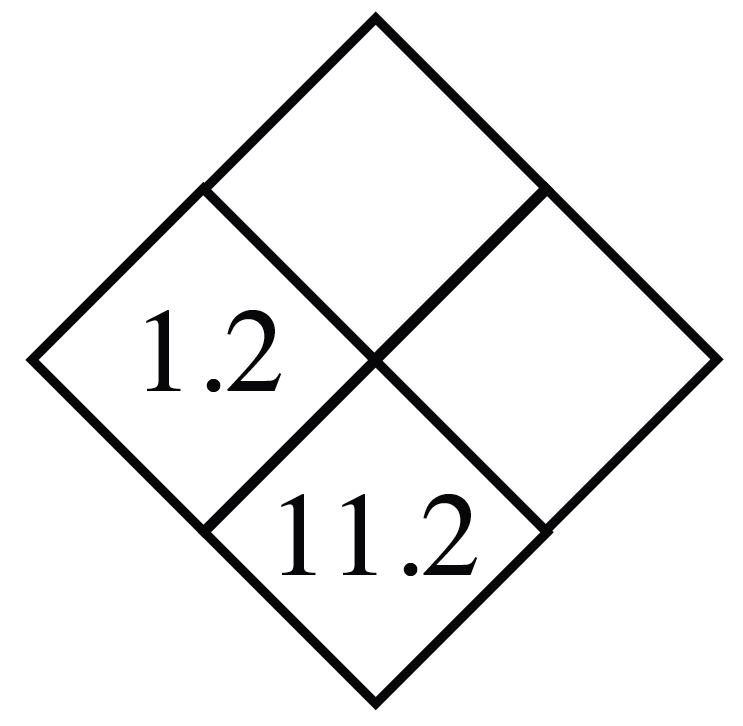$1.2+?=11.2$

1.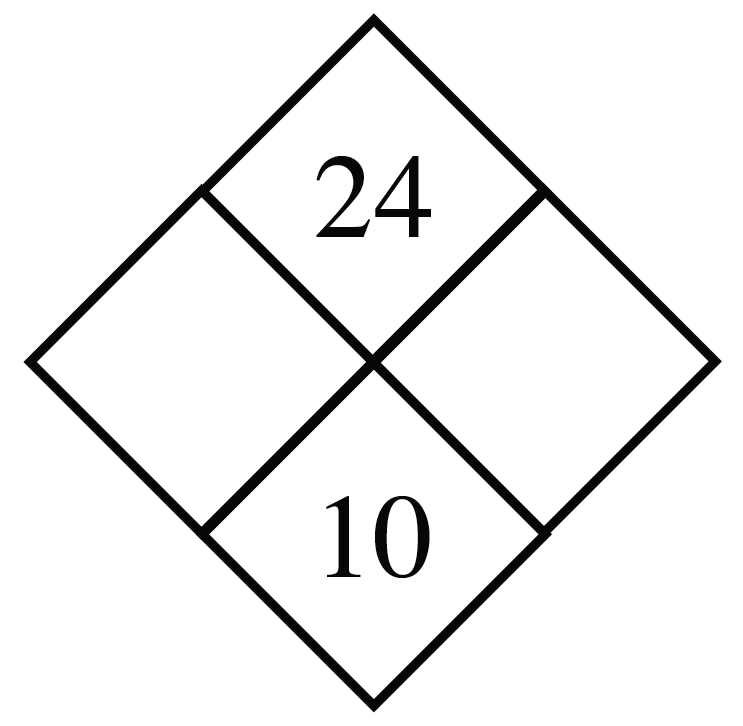What two numbers multiplied equal $24$, but equal $10$ when added together?